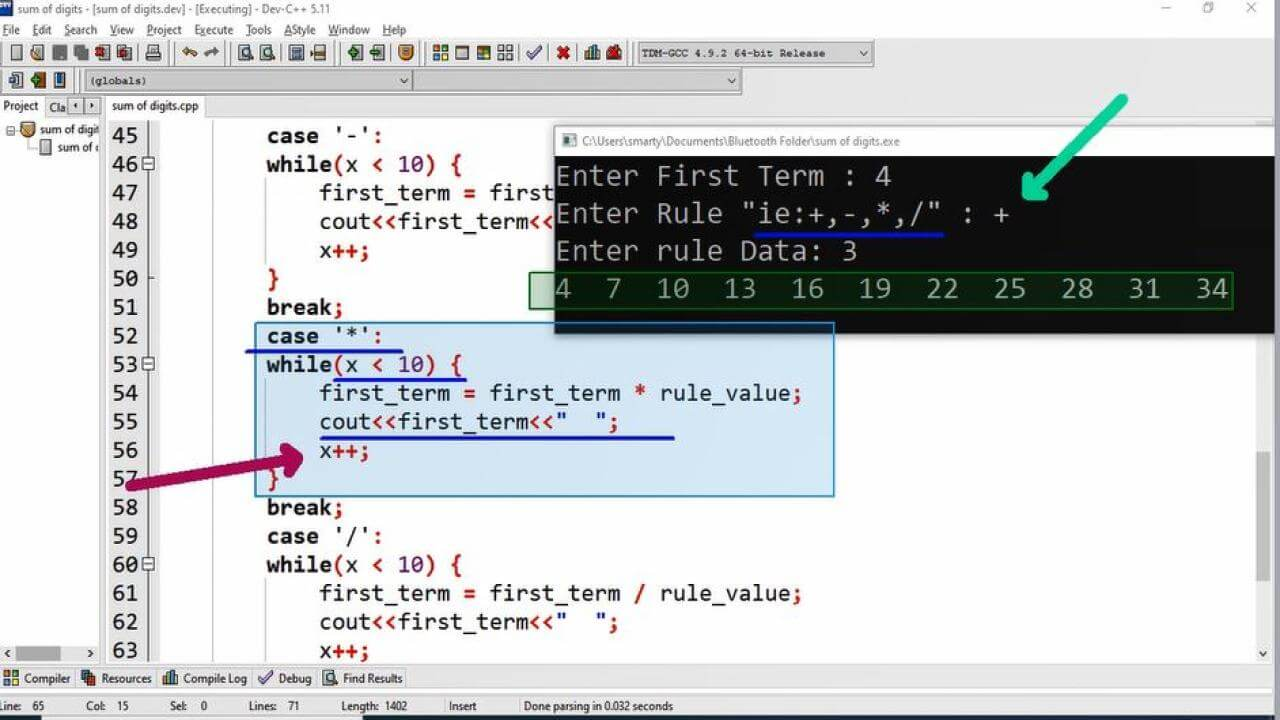# Arithmetic Sequence Program Example Using CPP

## Defination:

Arithmetic sequence is the series of number with some arithmetic difference. This sequence is aslo called arithmetic progression. Here first term and rule must be known in advance.

## Source Code:

#include <iostream>

// using standard namespace
using namespace std;

/*
This program will print
first 10 numbers from
the defined arithmetic
sequence.
*/

int main(int argc, char** argv) {

// variable declarations
float first_term; // used to store first term
char rule; // arithmetic operator

// used to add or subtract some value
int rule_value;
int x; //coutnter variable
// taking input
cout<<"Enter First Term : ";
cin>>first_term;
cout<<"Enter Rule \" ie:+,-,*,/ \" : ";
cin>>rule;
cout<<"Enter rule Data: ";
cin>>rule_value;

// printing first term
cout<<first_term<<"  ";

// Calculating and printing next 9 numbers
switch(rule) {
case '+':
while(x < 10) {
// calculating next coming terms.
first_term = first_term + rule_value;
// displaying next terms
cout<<first_term<<"  ";
x++; // incrementing counter variable
}
break;
case '-':
while(x < 10) {
first_term = first_term - rule_value;
cout<<first_term<<"  ";
x++;
}
break;
case '*':
while(x < 10) {
first_term = first_term * rule_value;
cout<<first_term<<"  ";
x++;
}
break;
case '/':
while(x < 10) {
first_term = first_term / rule_value;
cout<<first_term<<"  ";
x++;
}
break;
default:
cout<<".......Invalid Rule"<<endl;
}

return 0;
}

## Output:## Working:

In this program example we use while loop and switch statement. First of all we have to declare all necessary variables according to problem defination. The list of variables along with their description/use is as provided.

 Sr. Identifier Data Type Description 1 first_term float/double This variable is used to get input from user the first term of the sequence. 2 rule char rule variable will take single character input. Which may be any valid arithmetic operator. 3 rule_value int This variable will hold the value for generating upcoming values using the rule. 4 x int x is used as counter variable for iterations.

After complete declarations we take input as required to generate sequence.

• First Term
• Rule
• Rule Data Value

Here we use switch statement for checking different rules may input from the user. We can also implement this logic by if else statement if we are convinient with using if else if statement. In each case statement of switch we print series of 10 number using while loop and cout statement.

Before using  switch statement we print print nmber of series, which is taken from the user.

In each case statement while loop will print next 9 successive numbers of arithmetic sequence. Because first number is already displayed before switch statement. This algorithm is implemented only for +, -, * and /. So that if user input any operator except these the default statement will execute. That invalid operator. We can also change condition of while loop if we want to print more numbers.